# 彩色图像处理

Posted by jjx on December 27, 2016

#### 彩色基础

• 光源一一照射光的谱性质或谱能量分布．
• 物体－一被照射物体的反射性质．
• 成像接收器（眼睛或成像传感器）一一光谱能量吸收性质

(1）辐射率是从光源流出能量的总量， 通常用瓦特(W）度量；
(2）光强用流明度量，它给出了观察者从光源接收的能量总和的度量：
(3）亮度是彩色强度概念的具体化。它实际上是一个难以度量的主关描绘子．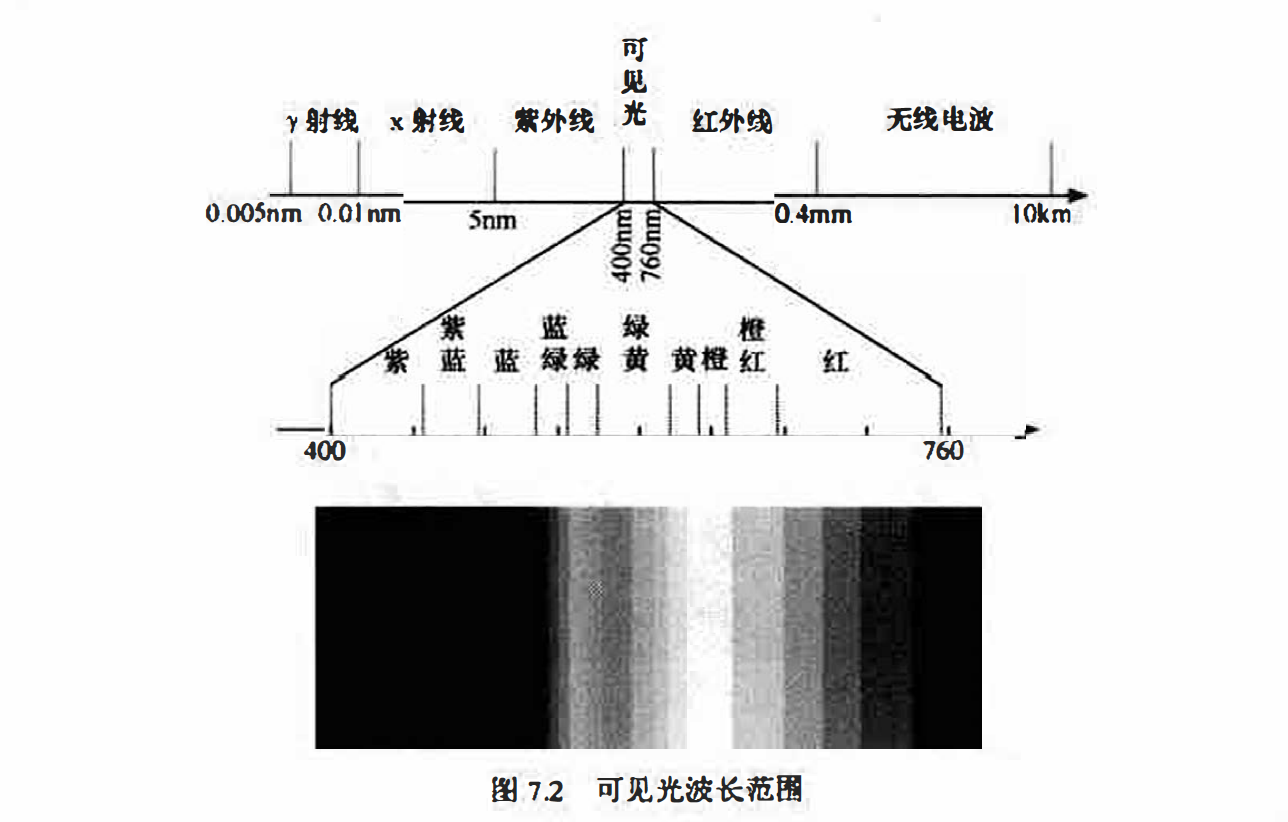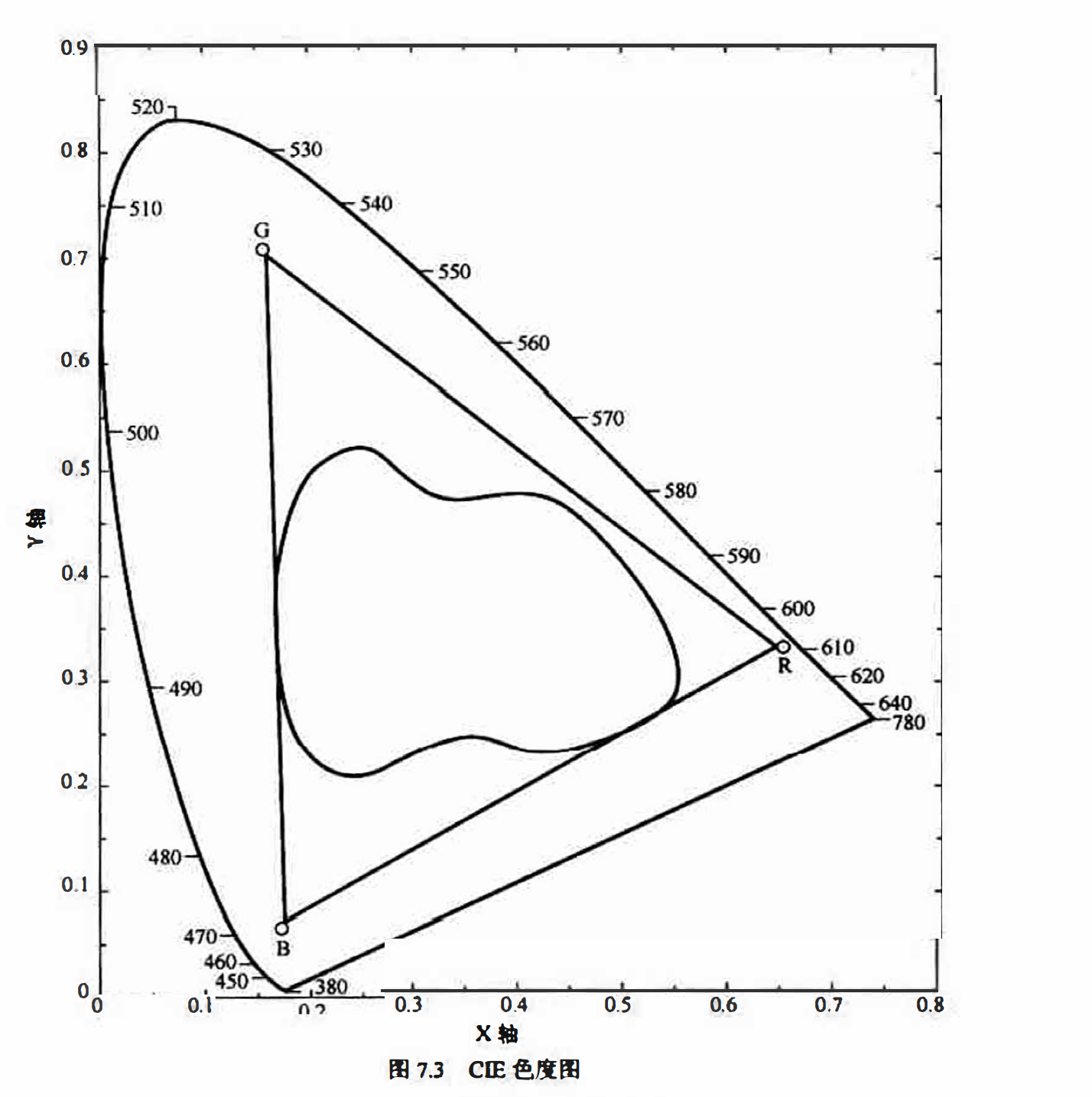• 计算机中的任何颜色都可以由3种颜色按不同比例混合而成；而每种颜色也都可以分解成三种基本颜色．
• 三原色之间相互独立，任何一种颜色都不能由其余两种颜色组成．
• 混合色的饱和度由3种颜色的比例来决定 混合色的亮度为3种颜色的亮度之和．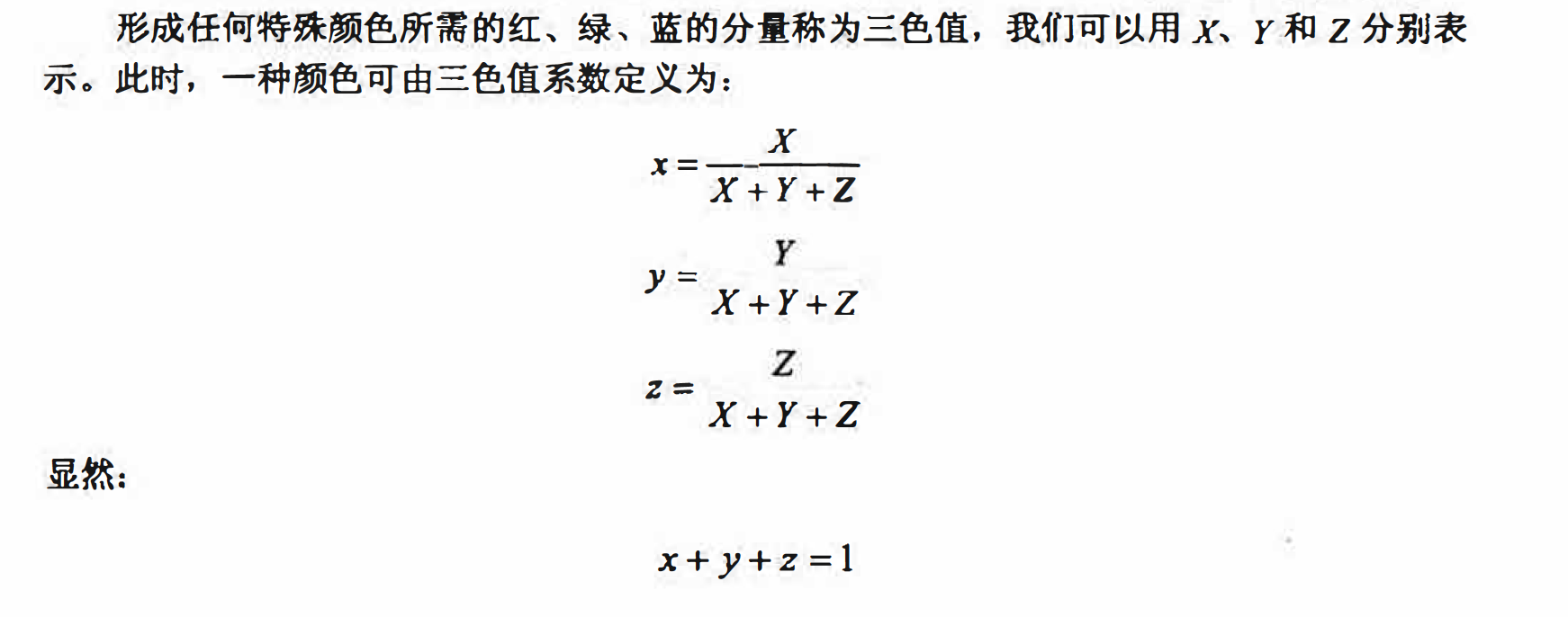### 彩色模型

#### RGB模型

RGB模型是工业界的一种颜色标准． 是通过对红（Red）、绿（Green）、蓝(Blue)3种颜色亮度的变化以及它们相互之间的叠加来得到各种各样的颜色的。该标准儿乎包括了人类视觉所能感知的所有颜色，是目前运用最广的颜色模型之一。

RGB彩色空间对应的坐标系统是如图7.4所示的立方体． 红、绿和蓝位手立方体的3个顶点上：青、深红和黄位于另外3个顶点上：黑色在原点处， 而白色位于距离原点最远的顶点处， 灰度等级就沿这两点连线分布： 不同的颜色处于立方体外部和内部， 因此可以用一个3维向量来表示。例如，在所有颜色均己归一化到（O, 1）的情况下，蓝色可表示为（O, 0, 1),而灰色可由向量（0.5, 0.5, 0.5）来表示。 在RGB模型中，3个图像分量组成了所要表示的图像，而每一个分量图像都是其原色图像，如图7.5所示。当送入RGB监视器时，这3个分量图像便在屏上混合产生一幅合成彩色图像：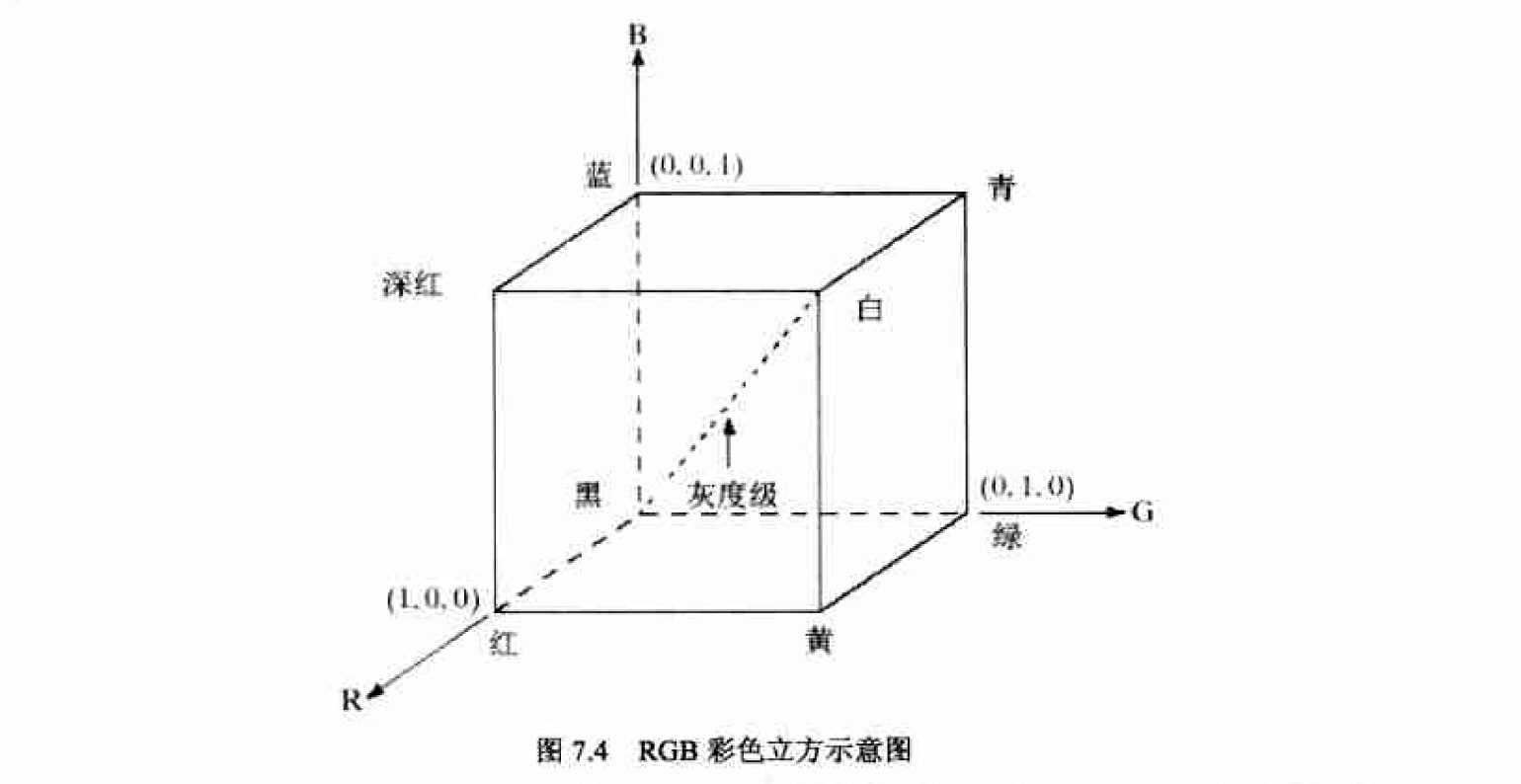在RGB空间中，用以表示每一像素的比特数叫做像素深度。RGB图像的3个红、绿、 蓝分量图像都是一幅8比特图像，每一个彩色像素有24比特深度。因此，全彩色图像常用来定义24比特的彩色图像，颜色总数是$(2^8)^3=16777216$

matlab实现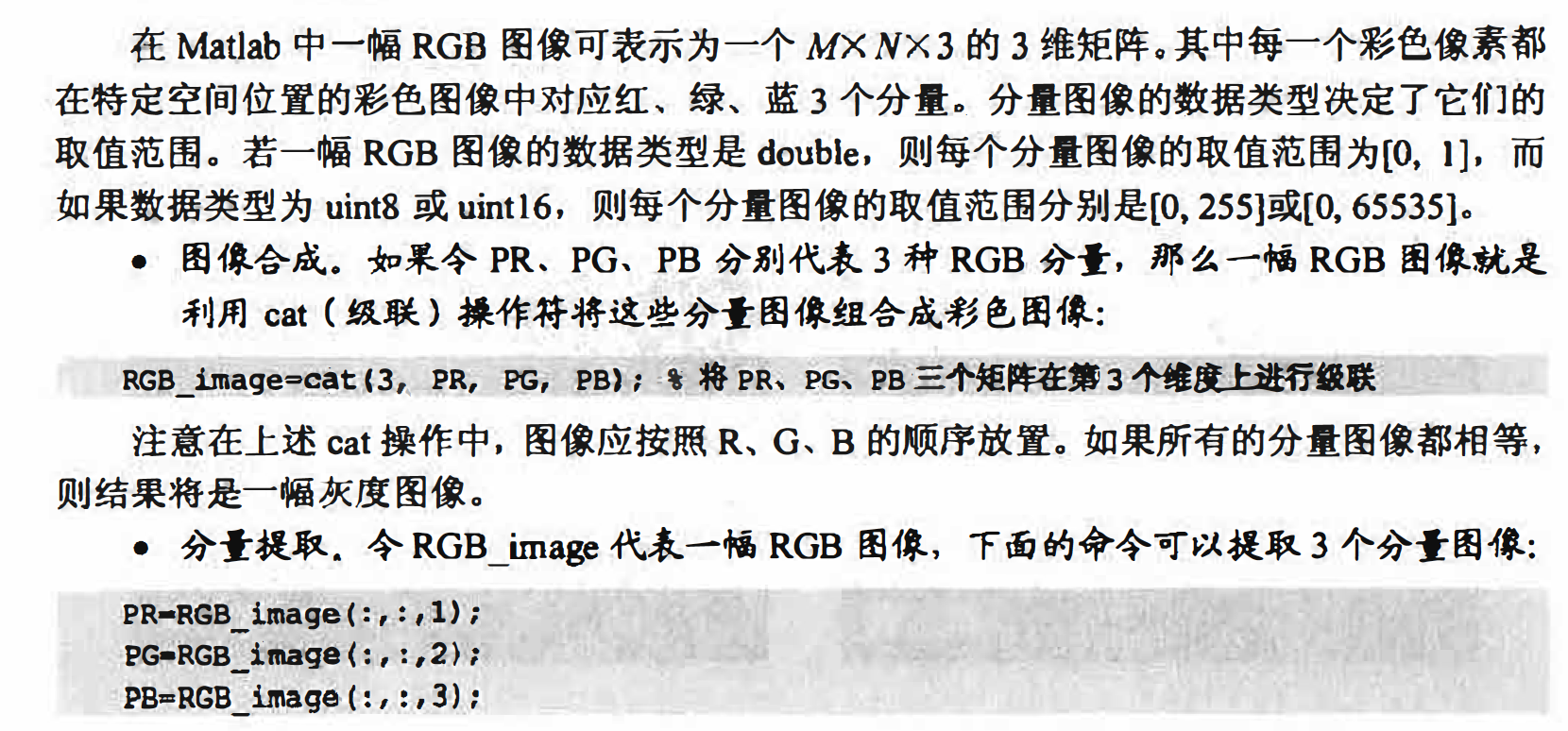#### CMY、CMYK模型

CMY模型（Cyan、Magenta、Yellow）是采用青、品红、黄色3种基本原色按一定比例合成颜色的方法。由于色彩的显示不是直接来自于光线的色彩，而是由光线被物体吸收掉一部分之后反射回来的剩余光线产生，因此CMY模型又称为减色法混色模型。当光线都被吸收时成为黑色，都被反射时成为白色．

CMYK模型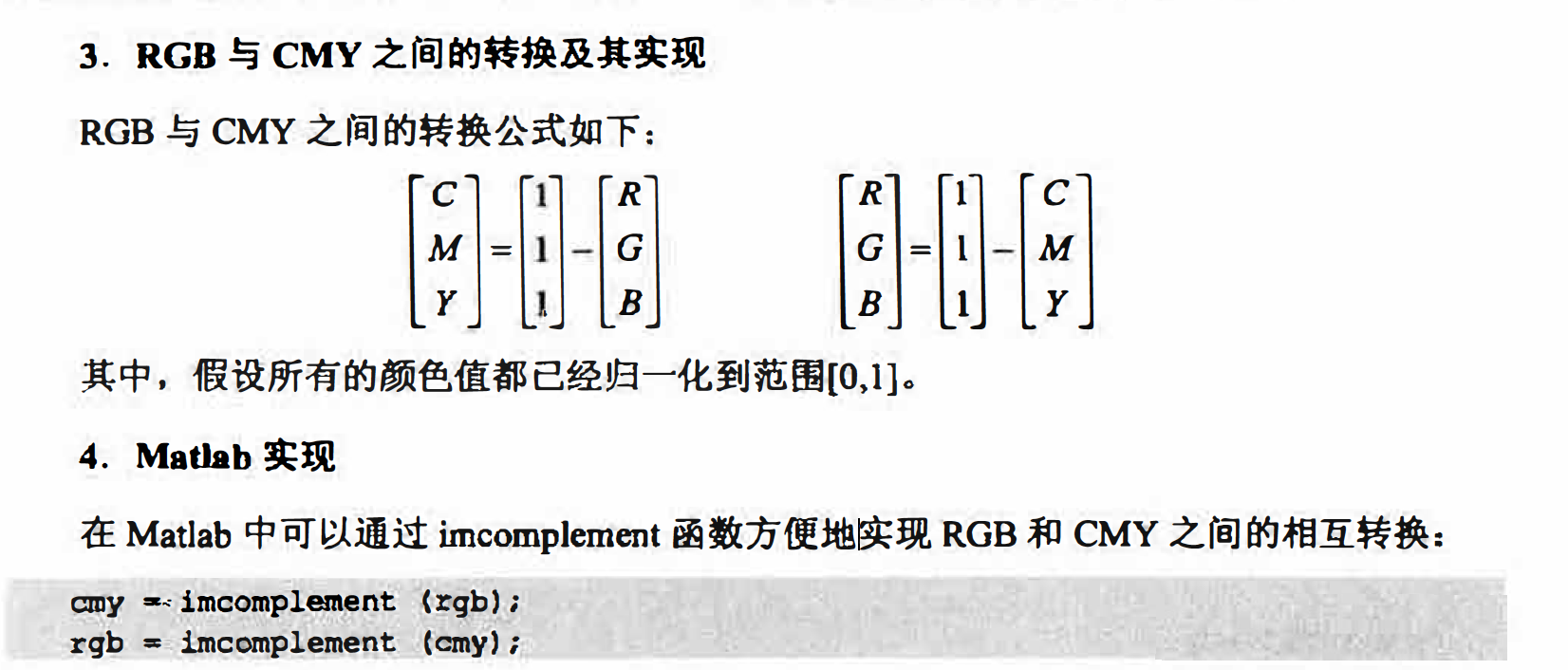#### HSI模型

HSI模型是从人的视觉系统出发，直接使用颜色三要素色调（Hue）、饱和度（Saturation) 和亮度(intensity，有时也翻译作密度或灰度〉来描述颜色.

• 亮度是指人眼感觉光的明暗程度．光的能量越大，亮度越大．
• 色调是彩色最重要的属性，决定颜色的本质，由物体反射光线中占优势的波长来决定． 不同的波长产生不同的颜色感觉，我们叫来一种颜色为红、橙、黄，这就是说我们在规定一种色调．
• 饱和度是指颜色的深浅和浓淡程度，饱和度越高，颜色越深．饱和度的深浅和白色的 比例有关，白色所占比例越高，饱和度越低．

HSI彩色空间可以用一个圆锥空间模型来描述， 如图7.8所示。我们通常把色调和饱和度统称为色度， 用来表示颜色的类别与深浅程度。在图中 四锥中间的横截面圆就是色度圆， 而圆锥向上或向下延伸的便是亮度分量的表示．

HSI彩色空间和RGB 彩色空间只是同一物理量的不同表示法， 它们之间存在着转换关系． 下面将介绍RGB到到HSI的彩色转换和HSI到RGB的彩色转换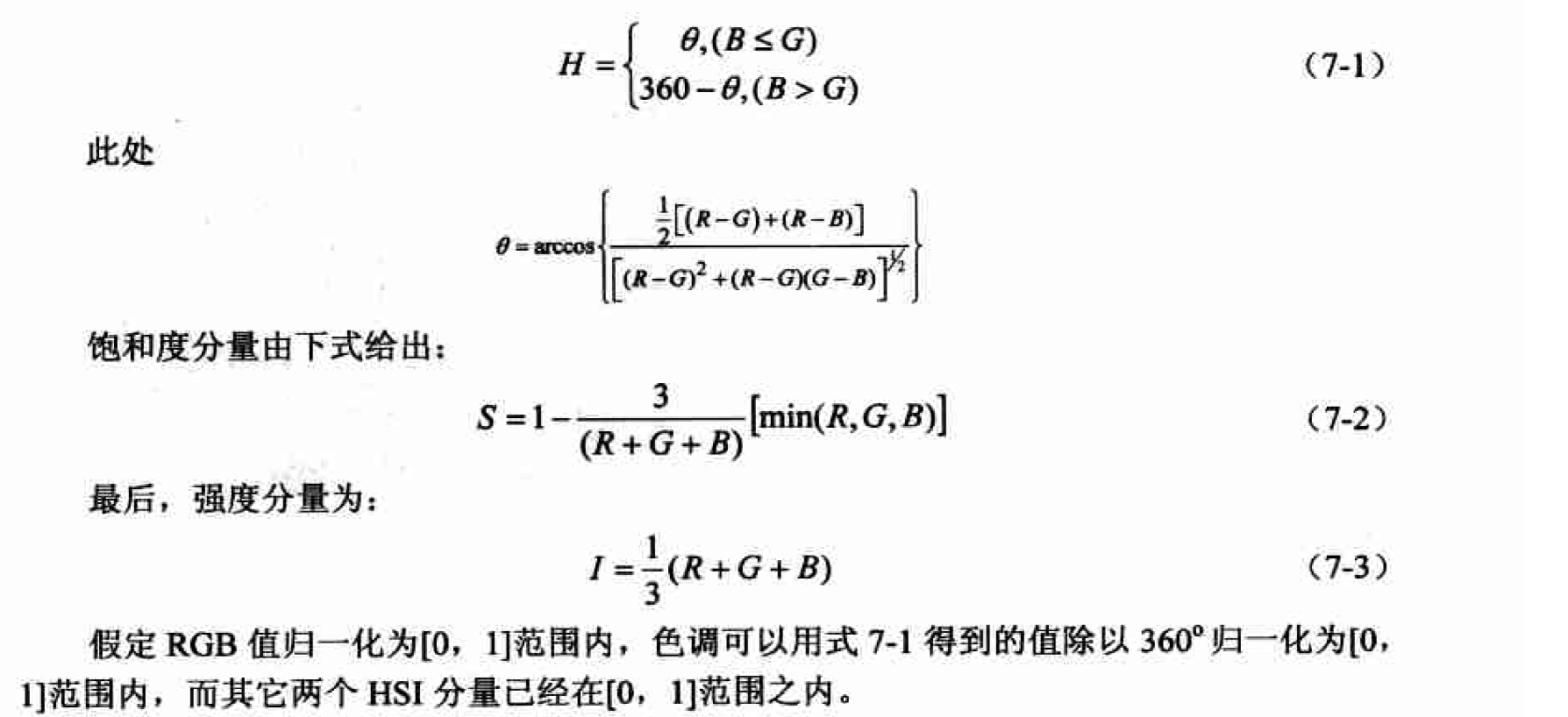matlab实现

function hsi = rgb2hsi(rgb)
% hsi = rgb2hsi(rgb)把一幅RGB图像转换为HSI图像，
% 输入图像是一个彩色像素的M×N×3的数组，
% 其中每一个彩色像素都在特定空间位置的彩色图像中对应红、绿、蓝三个分量。
% 假如所有的RGB分量是均衡的，那么HSI转换就是未定义的。
% 输入图像可能是double（取值范围是[0, 1]），uint8或 uint16。
%
% 输出HSI图像是double，
% 其中hsi(:, :, 1)是色度分量，它的范围是除以2*pi后的[0, 1]；
% hsi(:, :, 2)是饱和度分量，范围是[0, 1]；
% hsi(:, :, 3)是亮度分量，范围是[0, 1]。

% 抽取图像分量
rgb = im2double(rgb);
r = rgb(:, :, 1);
g = rgb(:, :, 2);
b = rgb(:, :, 3);

% 执行转换方程
num = 0.5*((r - g) + (r - b));
den = sqrt((r - g).^2 + (r - b).*(g - b));
theta = acos(num./(den + eps)); %防止除数为0

H = theta;
H(b > g) = 2*pi - H(b > g);
H = H/(2*pi);

num = min(min(r, g), b);
den = r + g + b;
den(den == 0) = eps; %防止除数为0
S = 1 - 3.* num./den;

H(S == 0) = 0;

I = (r + g + b)/3;

% 将3个分量联合成为一个HSI图像
hsi = cat(3, H, S, I);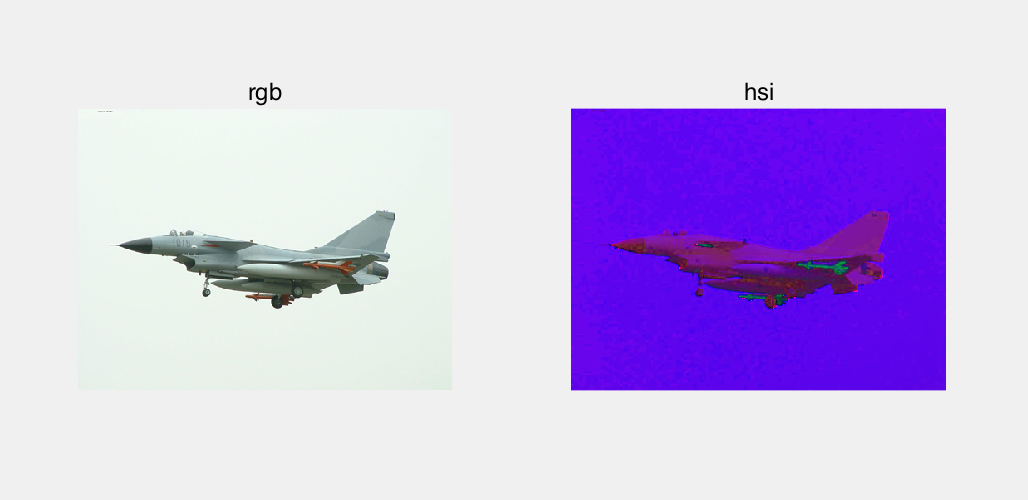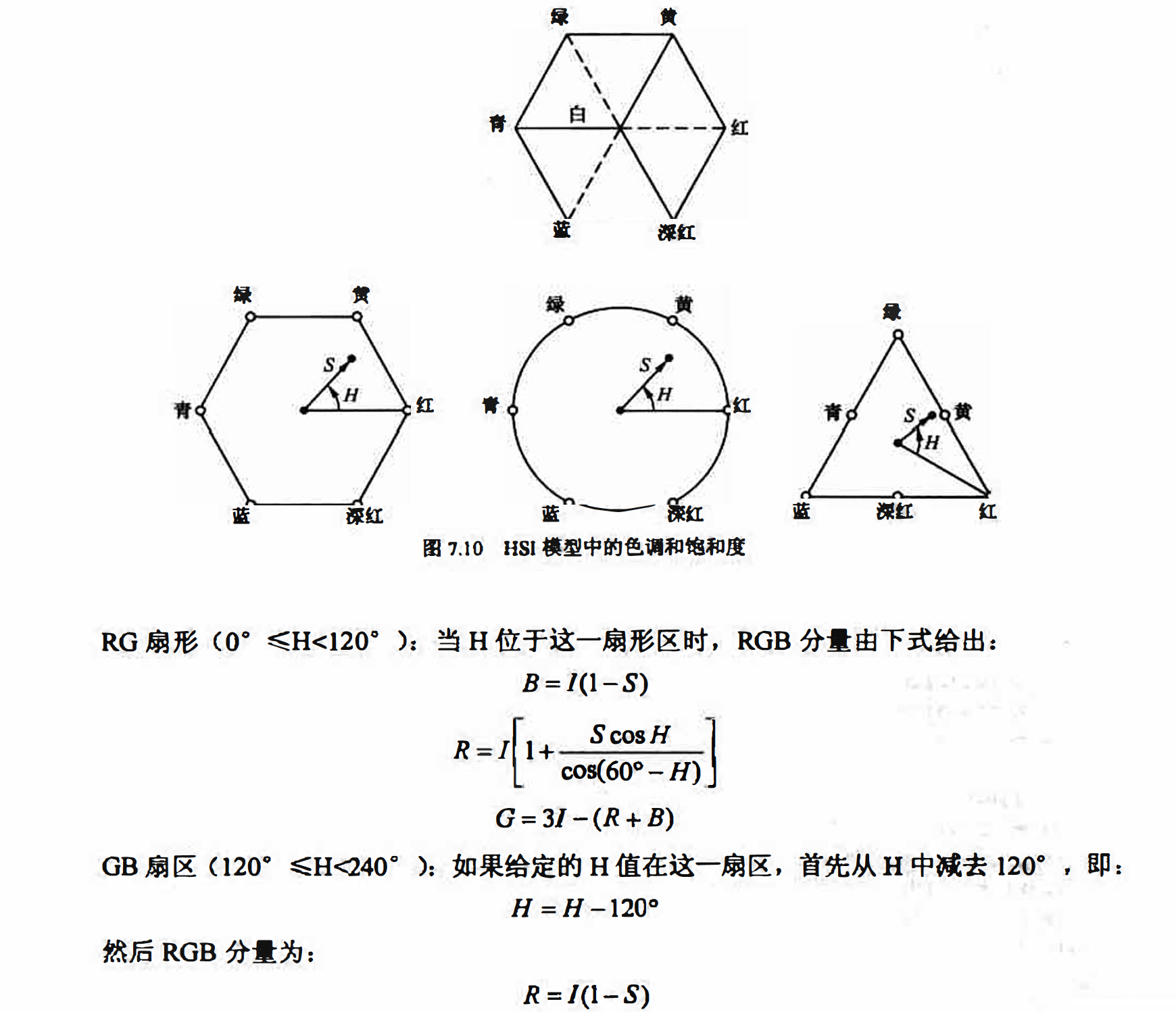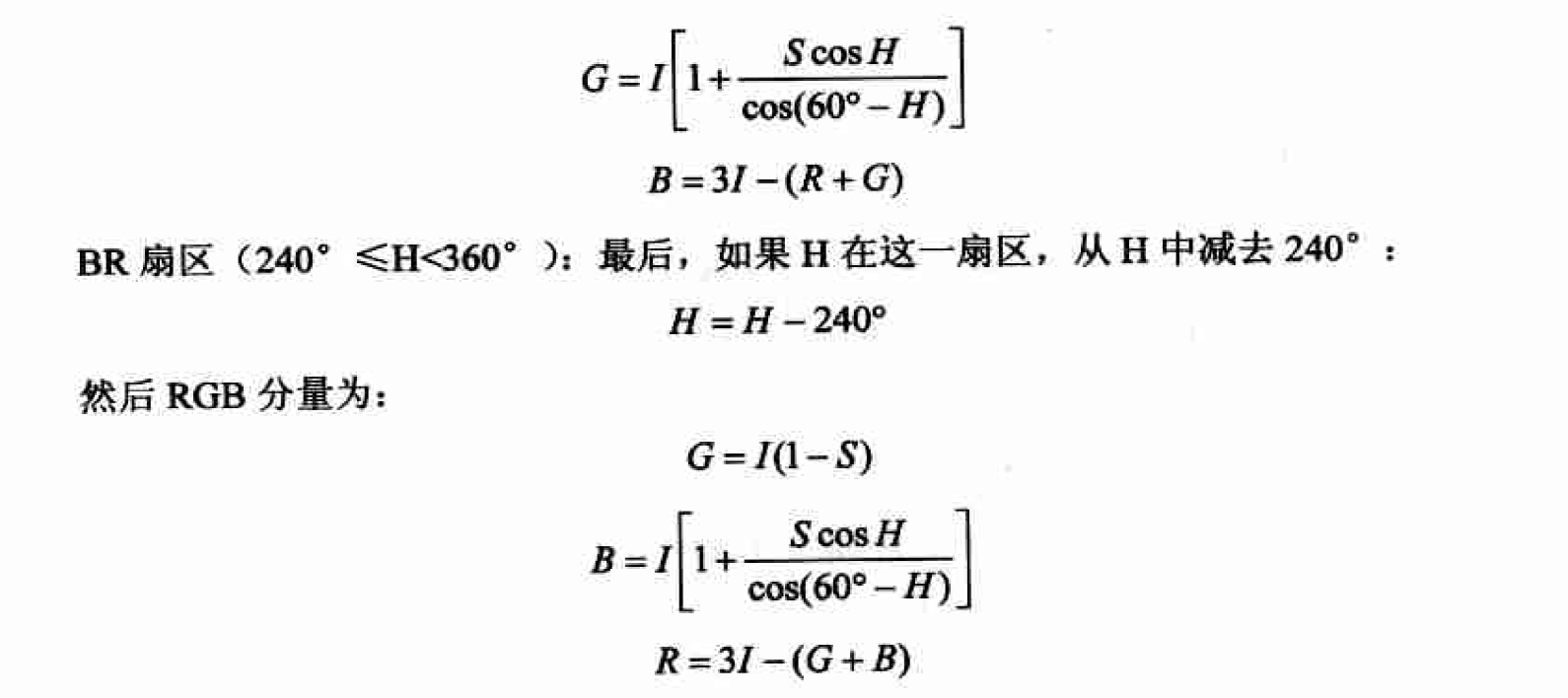matlab实现

function rgb = hsi2rgb(hsi)
% rgb = hsi2rgb(hsi)把一幅HSI图像转换为RGB图像，
% 其中hsi(:, :, 1)是色度分量，它的范围是除以2*pi后的[0, 1]；
% hsi(:, :, 2)是饱和度分量，范围是[0, 1]；
% hsi(:, :, 3)是亮度分量，范围是[0, 1]。
%
% 输出图像分量：
% rgb(:, :, 1)为红；
% rgb(:, :, 2)为绿；
% rgb(:, :, 3)为蓝。

% 抽取图像分量
hsi = im2double(hsi);
H = hsi(:, :, 1) * 2 * pi;
S = hsi(:, :, 2);
I = hsi(:, :, 3);

% 执行转换方程
R = zeros(size(hsi, 1), size(hsi, 2));
G = zeros(size(hsi, 1), size(hsi, 2));
B = zeros(size(hsi, 1), size(hsi, 2));

% RG扇形(0 <= H < 2*pi/3)
idx = find( (0 <= H) & (H < 2*pi/3));
B(idx) = I(idx) .* (1 - S(idx));
R(idx) = I(idx) .* (1 + S(idx) .* cos(H(idx)) ./ ...
cos(pi/3 - H(idx)));
G(idx) = 3*I(idx) - (R(idx) + B(idx));

% BG扇形(2*pi/3 <= H < 4*pi/3)
idx = find( (2*pi/3 <= H) & (H < 4*pi/3) );
R(idx) = I(idx) .* (1 - S(idx));
G(idx) = I(idx) .* (1 + S(idx) .* cos(H(idx) - 2*pi/3) ./ ...
cos(pi - H(idx)));
B(idx) = 3*I(idx) - (R(idx) + G(idx));

% BR扇形
idx = find( (4*pi/3 <= H) & (H <= 2*pi));
G(idx) = I(idx) .* (1 - S(idx));
B(idx) = I(idx) .* (1 + S(idx) .* cos(H(idx) - 4*pi/3) ./ ...
cos(5*pi/3 - H(idx)));
R(idx) = 3*I(idx) - (G(idx) + B(idx));

% 将3个分量联合成为一个RGB图像
rgb = cat(3, R, G, B);
rgb = max(min(rgb, 1), 0);


### 全彩色图像处理基础

(1）对3个平面分量单独处理，然后将分别处理过的3个分量合成彩色图像。对每个分量的处理技术可以应用到对灰度图像处理的技术上。但是这种通道式的独立处理技术忽略了通道间的相互影响。
(2）直接对彩色像素进行处理。因为全彩色图像至少有3个分量，彩色像素实际上是一个向量。直接处理就是同时对所有分量进行无差别的处理．这时彩色图像的 3个分量用向量形式表示，即对彩色图像上任一点的像素 c(x,y)，有：
c(x,y)= [R(x,y);G(x,y);B(x,y)]

#### 彩色补偿及其Matlab实现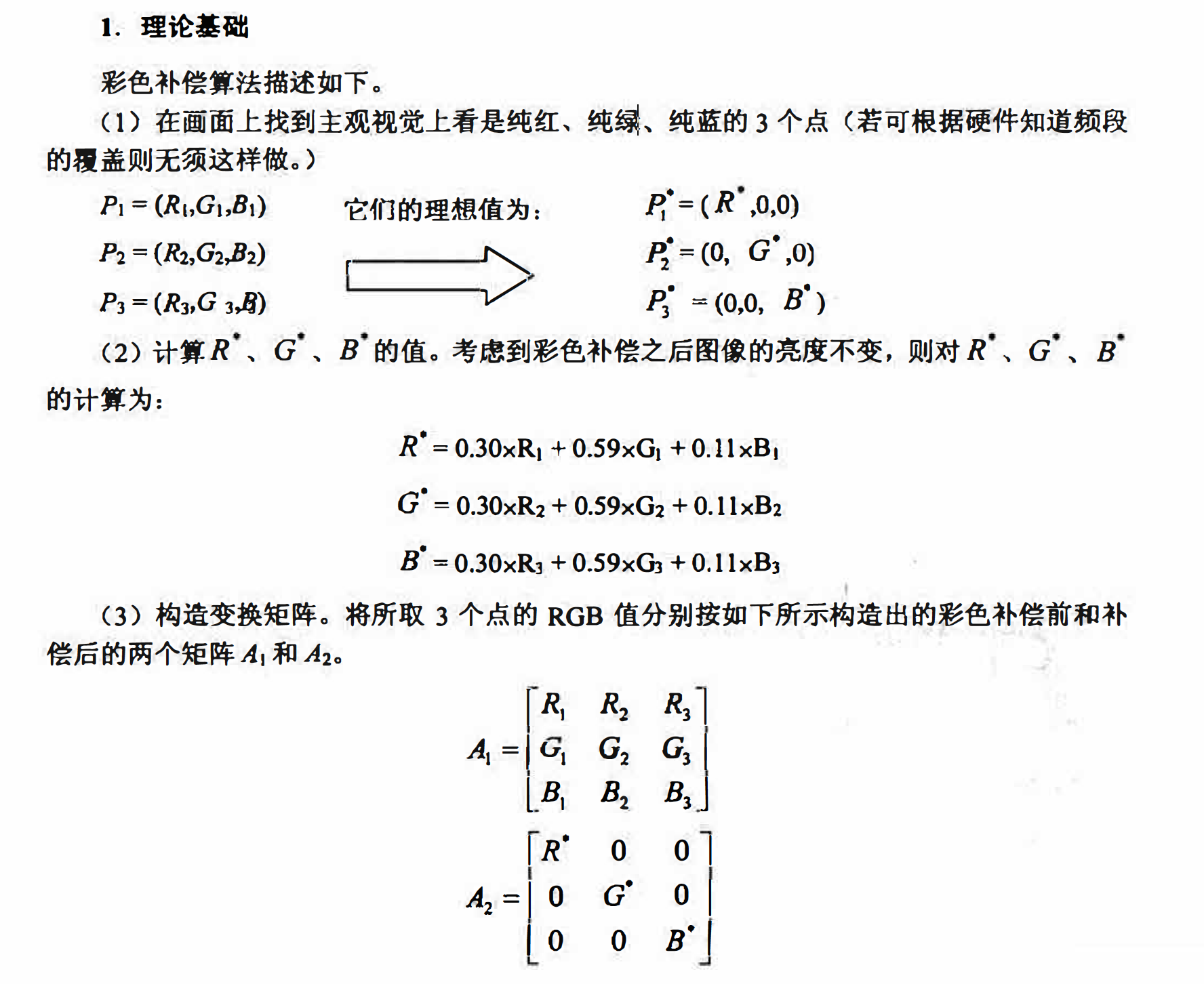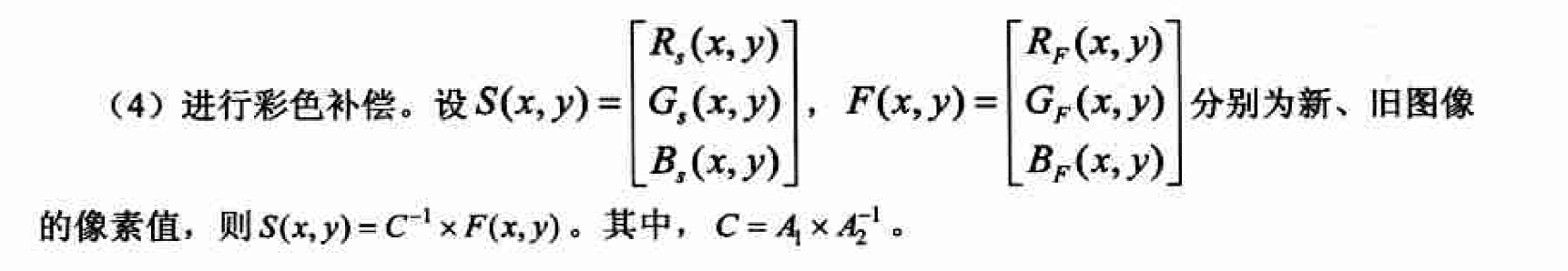matlab实现

% compensate.m
% 彩色补偿
subplot(1,2,1);
imshow(uint8(im));
title('原始图');
[m,n,p]=size(im);
[h1,k1]=min(255-im(:,:,1)+im(:,:,2)+im(:,:,3));
[j1,minx]=min(h1);
i1=k1(j1);%提取图像中最接近红色的点，其在im中的坐标为i1,j1
r1=im(i1,j1,1);
g1=im(i1,j1,2);
b1=im(i1,j1,3);
R=0.30*r1+0.59*g1+0.11*b1;

[h2,k2]=min(255-im(:,:,2)+im(:,:,1)+im(:,:,3));
[j2,minx]=min(h2);
i2=k2(j2);%提取图像中最接近绿色的点，其在im中的坐标为i2,j2
r2=im(i2,j2,1);
g2=im(i2,j2,2);
b2=im(i2,j2,3);
G=0.30*r2+0.59*g2+0.11*b2;

[h3,k3]=min(255-im(:,:,3)+im(:,:,1)+im(:,:,2));
[j3,minx]=min(h3);
i3=k3(j3);%提取图像中最接近蓝色的点，其在im中的坐标为i3,j3
r3=im(i3,j3,1);
g3=im(i3,j3,2);
b3=im(i3,j3,3);
B=0.30*r3+0.59*g3+0.11*b3;

A1=[r1 r2 r3
g1 g2 g3
b1 b2 b3];
A2=[R 0 0
0 G 0
0 0 B];
C=A1*inv(A2);

for i=1:m
for j=1:n

imR=im(i,j,1);
imG=im(i,j,2);
imB=im(i,j,3);
temp=inv(C)*[imR;imG;imB];
S(i,j,1)=temp(1);
S(i,j,2)=temp(2);
S(i,j,3)=temp(3);
end
end
S=uint8(S);
subplot(1,2,2);
imshow(S);
title('补偿后');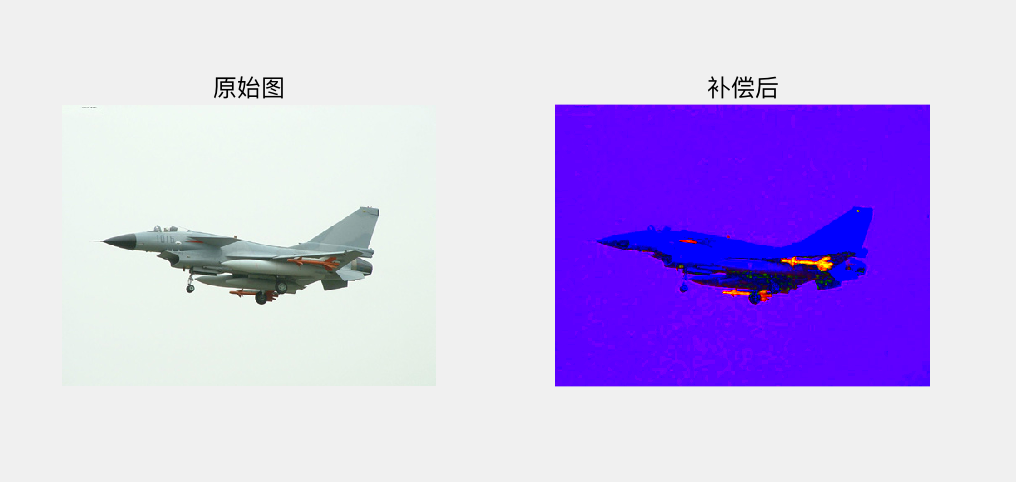#### 彩色平衡及其Matlab实现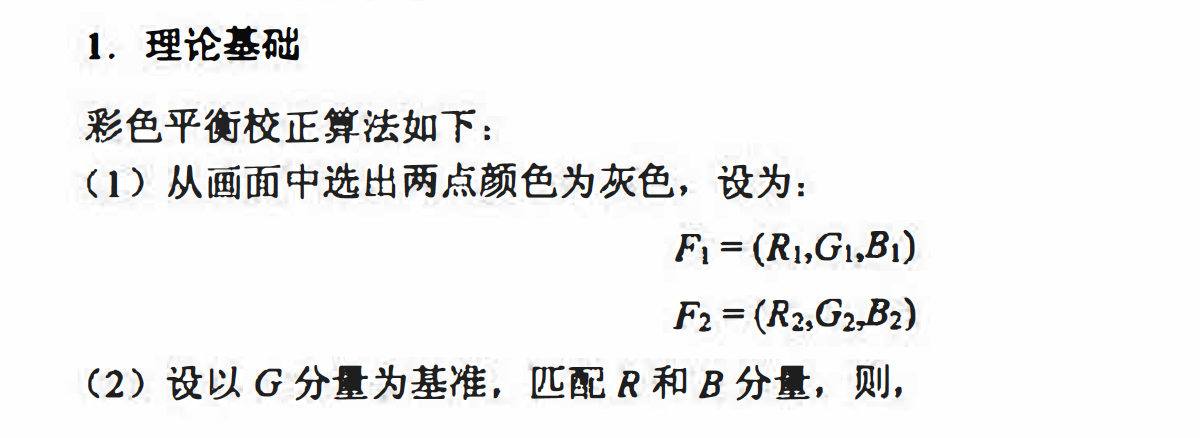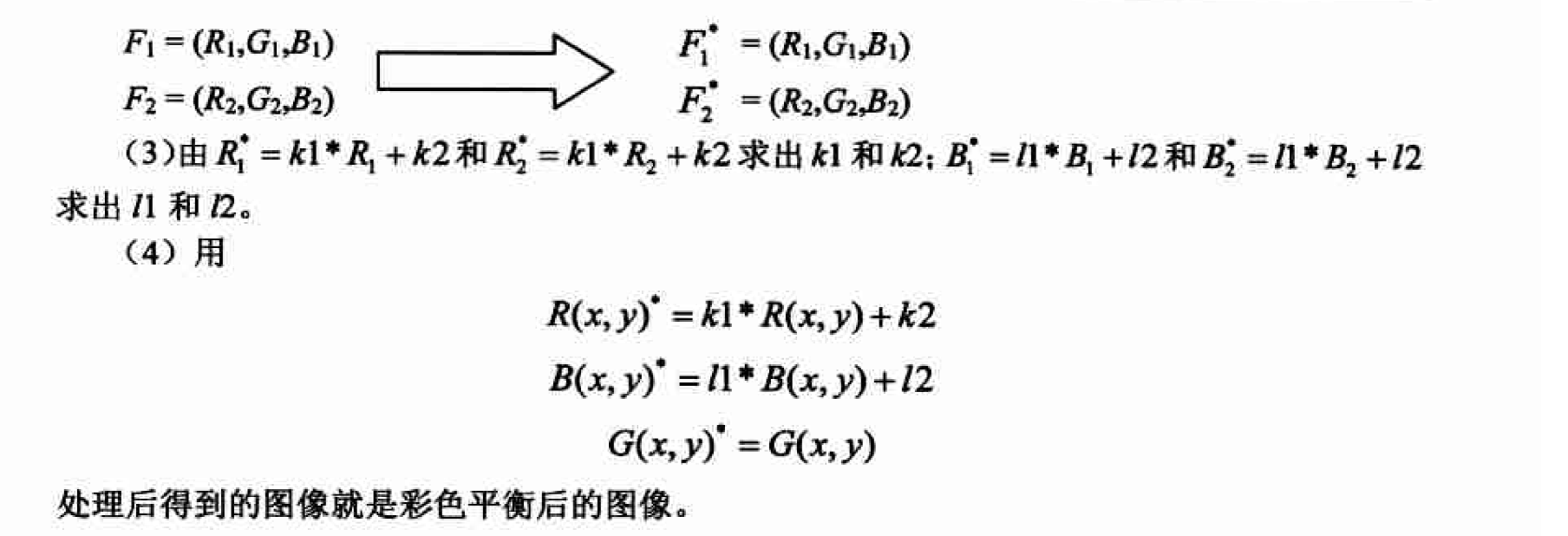matlab实现

% balance.m
% 彩色平衡

[m,n,p]=size(im);
F1=im(1,1,:);
F2=im(1,2,:);
F1_(1,1,1)=F1(:,:,2);
F1_(1,1,2)=F1(:,:,2);
F1_(1,1,3)=F1(:,:,2);
F2_(1,1,1)=F2(:,:,2);
F2_(1,1,2)=F2(:,:,2);
F2_(1,1,3)=F2(:,:,2);
K1=(F1_(1,1,1)-F2_(1,1,1))/(F1(1,1,1)-F2(1,1,1));
K2=F1_(1,1,1)-K1*F1(1,1,1);
L1=(F1_(1,1,3)-F2_(1,1,3))/(F1(1,1,3)-F2(1,1,3));
L2=F1_(1,1,3)-L1*F1(1,1,3);
for i=1:m
for j=1:n
new(i,j,1)=K1*im(i,j,1)+K2;
new(i,j,2)=im(i,j,2);
new(i,j,3)=L1*im(i,j,3)+L2;
end
end
im=uint8(im);
new=uint8(new);
subplot(1,2,1);
imshow(im);
title('原始图');
subplot(1,2,2);
imshow(new);
title('平衡后');